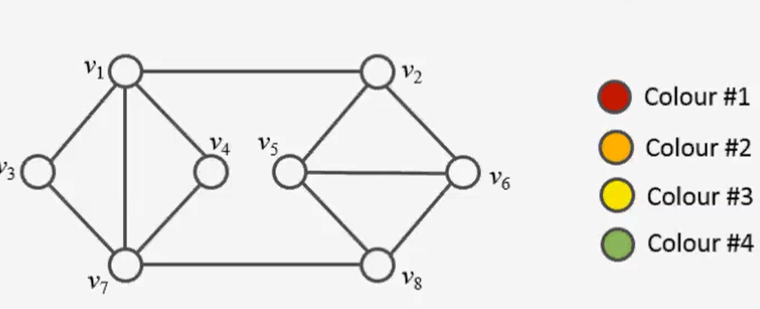## Vertex Coloring using ILPIn this post I’ll show you how to solve the vertex coloring problem to optimality using linear programming. Since the problem is NP-complete, there is no algorithm which can solve any problem instance in deterministic polynomial time. In a former post, I used constraint programming to find an optimal (minimal) vertex coloring. A popular greedy… Read more Vertex Coloring using ILP